×

Search anything:

# Simplified: C++ STL Lists

#### Software Engineering C++Get this book -> Problems on Array: For Interviews and Competitive Programming

Reading time: 30 minutes | Coding time: 10 minutes

List, as the name suggests, is the container to store the homogeneous data (data of the same type). It allocates memory based on a non-contiguous scheme. As compared to arrays/vector, the C++ list has some trade-offs. Before going forward,if you don't know about linked lists, I would recommend you to do check out the article on the singly-linked list and doubly-linked list by Rahul Kumar.

Unlike arrays that provide O(1) lookup time, in lists, we have to traverse to that position to get the value, so it allows for O(n) lookup time. But the list has a significant advantage over arrays. Let's take an example to understand this. Consider you want to insert an element in the middle of the array.

## What do you think the time complexity for that would be?

We have to shift all the elements after that position to insert new element in that position. So it would take O(n) time.

That's right; it would be O(n), but with the lists, you can achieve the same task in the constant time provided that you have to iterator or position where the element is to be inserted. Now many would argue that to get to the position of insertion, we have to traverse the list, but we will see many cases where we have stored the iterator to the position beforehand and then voila! We can get our work done in constant time. Same way, deletion operation is performed, and we can delete the element at a specified position in continuous time.

Here's the quick animation to visualize the insertion in linked list.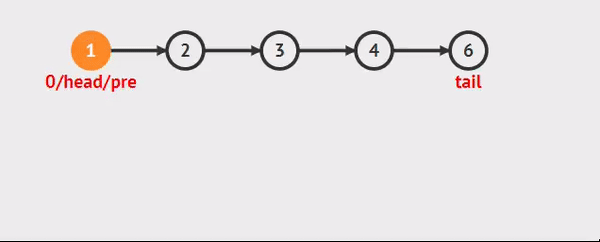By using the C++ STL list, you don’t have to worry about the implementation as it is pre implemented in the STL library, which is quite handy for c++ users. List containers are implemented as doubly-linked lists that means we can iterate in both the direction forward or backward.

``````template < class T, class Alloc = allocator<T> > class list;
``````

T: Type of the elememt to be stored.
Alloc: Type of the allocator used to define the storage allocation model.

Don’t be scared if you don’t know about allocator or template, this definition is just for knowledge and it has nothing to do with usage of the list.

# Here's the syntax to declare list:

``````list<int> mylist
``````

It tell the compiler to declare variable mylist of std:list type.

## Iterators

Let’s dive into the iterators provided by std:list. C++ provides the wide range of iterator with the lists and here I have listed them along with their function. Please note each of these methods have O(1) complexity:

1. begin(): Return iterator to beginning of the list.
2. end(): Return iterator to end of the list.
3. rbegin(): Return reverse iterator to reverse beginning.
4. rend(): Return reverse iterator to reverse end.
5. cbegin(): Return const_iterator to beginning.
6. cend(): Returns the cosntant iterator to the end of the list.
7. crbegin(): Return const_reverse_iterator to reverse beginning.
8. crend(): Return const_reverse_iterator to reverse end.

Here's the visualization: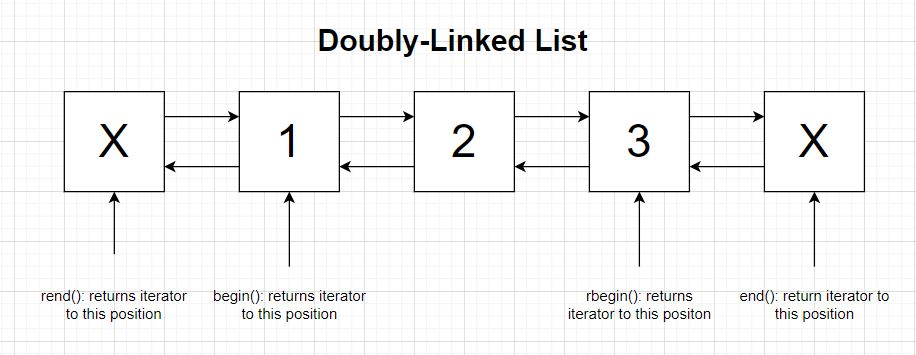The iterators come in use while traversing the list. C++ code to traverse the list:

``````#include<list>
#include <iostream>
using namespace std;
int main() {
list<int> mylist = {1, 2, 3, 4, 5};
for(auto itr = mylist.begin();itr!=mylist.end();++itr){
cout<<*itr<<"\n";
}
}
``````

From now onwards we will use this code snippet to understand other functions of the list.

## Capacity

Now we have capacity functions which tell about the size status of the list. These funtions also have O(1) complexity:

1. empty(): Checks whether the list is empty.
Eg: mylist.empty() returns false.
2. size(): Return the number of elements in the list.
Eg: mylist.size() returns 5

# Access Functions

C++ list does not provide element access in between the list, but we do have access to the front element and last element of the list, and be careful these are not the iterator. And again these have O(1) complexity.

1. front(): Returns the element at the beginning of the list. Eg: mylist.front() returns 1
2. back(): Return the element at the end of the list. Eg: mylist.() back returns 5

# Modifiers

Now we will talk about the main functions which helps us to modify the list.

1. assign(): Assign the given values to the list. Eg: mylist.assign(5,10) makes a list with 5 element with value 10. It has time complexity of O(n). O(n) Complexity
2. emplace_front(): Constructs and insert element at the beginning of the list. Eg: mylist.emplace_front(3) adds 3 at the front of the list. O(1) Complexity
3. emplace_back(): Constructs and insert element at the end of the list. Eg: >mylist.emplace_front(3) adds 3 at the end of the list. O(1) Complexity
4. push_front(): Insert element at the beginning of the list. Eg: mylist.push_front(9) adds 9 at the beginning of the list. O(1) Complexity
5. push_back(): Insert element at the end of the list. Eg: mylist.push_back(1) adds 1 at the end of the list. O(1) Complexity
6. pop_front(): Delete element at the beginning of the list. Eg: mylist.pop_front() deletes 1 from the list. O(1) Complexity
7. pop_back(): Delete element at the end of the list. Eg: mylist.pop_back() deletes 4 from the list. O(1) Complexity
8. insert(): Insert element at the specified position. Eg: mylist.insert(itr,3) inserts 3 at position specified by itr. O(1) Complexity
9. erase(): Delete element from specified position. Eg: mylist.erase(itr) delete element specified by itr. O(1) Complexity
10. clear(): Erase all the elements from list. Eg: mylist.clear() deletes all element from the mylist. O(n) Complexity

# Here's how push and pop works: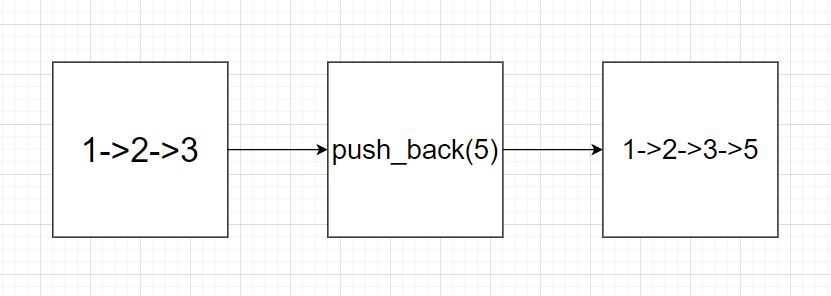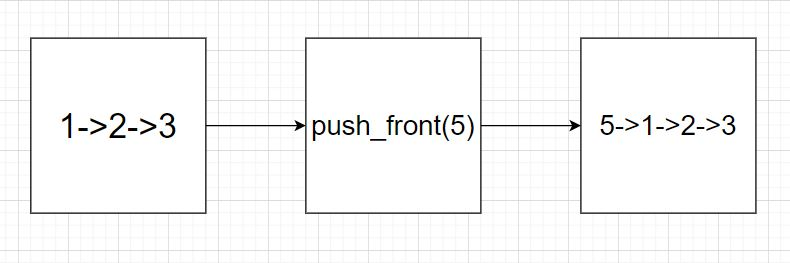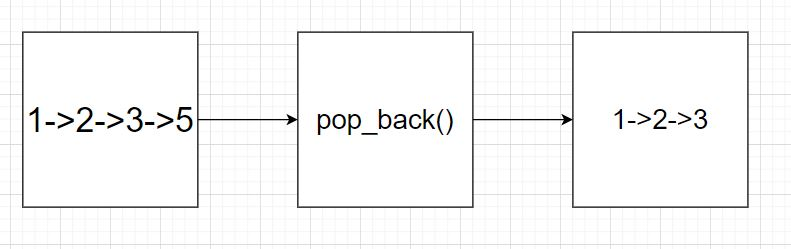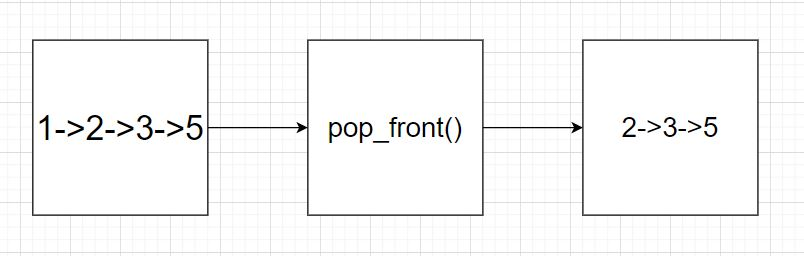# Operations

We have finished knowing about the modifiers in detail and now you know 80% about the std:list. Last type of functions are operations on list.

1. splice(): Transfer elements from one list to another. Eg: list1.splice(list1.end(),list2) transfer elements from list2 and append at list1. O(n) Complexity
2. remove(): Remove element with specified value. Eg: mylist.remove(2) removes 2 from the list. O(n) Complexity
3. sort(): Sort the list, by default in ascending order. Eg: mylist.sort() sorts the list. O(nlog(n)) Complexity
4. reverse(): Reverse the order of elements in the list. Eg: mylist.reverse() reverses the list and now content of list is [4,3,2,1]. O(n) Complexity

# Now here's how sort and reverse works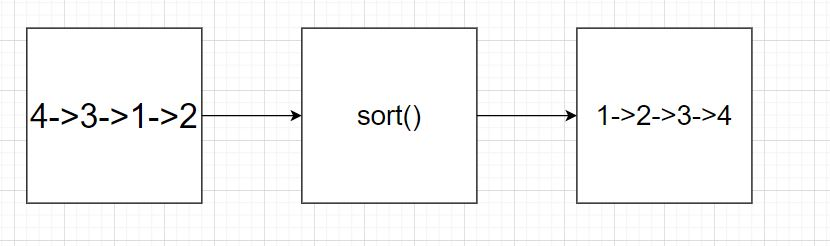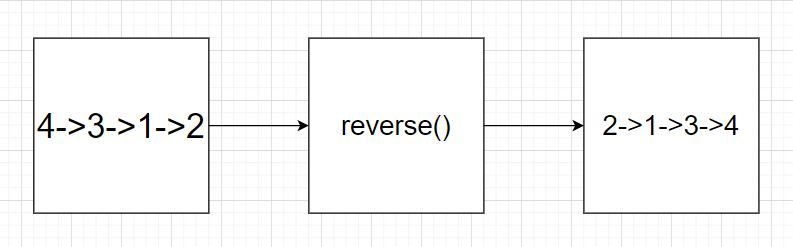# Problem Statement:

The function given accepts two different std:lists as its parameter. Your task is to complete the function so that it merges both the lists and return it back in ascending order.

``````list<int>& merge_and_sort(list<int> &l1,list<int> &l2){
}
``````

Pause here and think how can you use STL list functions to achieve this task.

I hope you have arrived at the solution, so here is the one way to do it.

``````list<int>& merge_and_sort(list<int> &l1,list<int> &l2){
l1.splice(l1.end(),l2);
sort(l1.begin(),l1.end());
return l1;
}
``````

# Applications

STL List is simply a implementation of doubly-linked list so std:list itself has no application except it saves the time to implement linked list. Here are some of the applications of linked list.

• It helps in implementing stacks and queues.
• It is used to dynamically allocate memory.
• Manipulation of polynomials by storing constants in the node of linked list
• It helps in representation of sparse matrix.